1. /
2. CBSE
3. /
4. Class 08
5. /
6. Mathematics
7. /
8. NCERT Solutions for Class...

# NCERT Solutions for Class 8 Maths Exercise 13.2### myCBSEguide App

Download the app to get CBSE Sample Papers 2023-24, NCERT Solutions (Revised), Most Important Questions, Previous Year Question Bank, Mock Tests, and Detailed Notes.

NCERT solutions for Class 8 Maths Direct and Inverse Proportions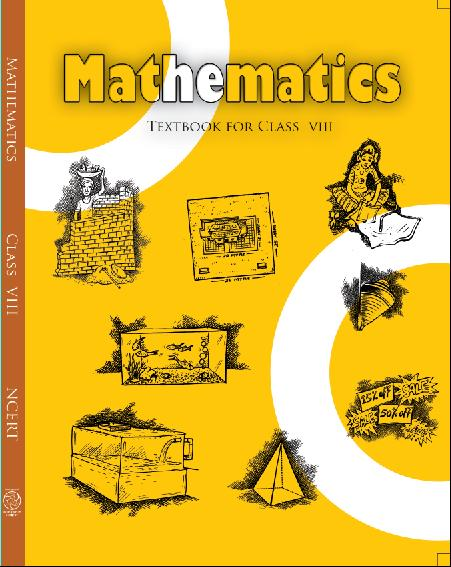## NCERT Solutions for Class 8 Maths Direct and Inverse Proportions

###### 1. Which of the following are in inverse proportion:

(i) The number of workers on a job and the time to complete the job.

(ii) The time taken for a journey and the distance travelled in a uniform speed.

(iii) Area of cultivated land and the crop harvested.

(iv) The time taken for a fixed journey and the speed of the vehicle.(v) The population of a country and the area of land per person.

Ans. (i) The number of workers and the time to complete the job is in inverse proportion

because less workers will take more time to complete a work and more workers will take less time to complete the same work.

(ii) Time and distance covered in direct proportion.

(iii) It is a direct proportion because more are of cultivated land will yield more crops.

(iv) Time and speed are inverse proportion because if time is less, speed is more.

(v) It is a inverse proportion. If the population of a country increases, the area of land per person decreases.

NCERT Solutions for Class 8 Maths Exercise 13.2

###### 2. In a Television game show, the prize money of Rs.1,00,000 is to be divided equally amongst the winners. Complete the following table and find whether the prize money given to an individual winner is directly or inversely proportional to the number of winners: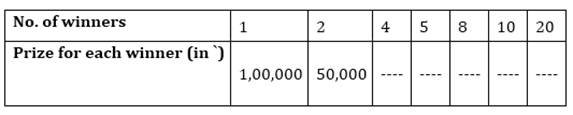Ans. Here number of winners and prize money are in inverse proportion because winners are increasing, prize money is decreasing.

When the number of winners are 4, each winner will get =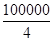= Rs. 25,000

When the number of winners are 5, each winner will get =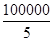= Rs. 20,000

When the number of winners are 8, each winner will get =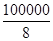= Rs. 12,500

When the number of winners are 10, each winner will get =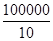= Rs. 10,000

When the number of winners are 20, each winner will get =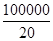= Rs.  5,000

NCERT Solutions for Class 8 Maths Exercise 13.2

###### 3. Rehman is making a wheel using spokes. He wants to fix equal spokes in such a way that the angles between any pair of consecutive spokes are equal. Help him by completing the following table: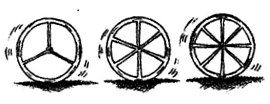(i) Are the number of spokes and the angles formed between the pairs of consecutive spokes in inverse proportion?

(ii) Calculate the angle between a pair of consecutive spokes on a wheel with 15 spokes.

(iii) How many spokes would be needed, if the angle between a pair of consecutive spokes is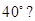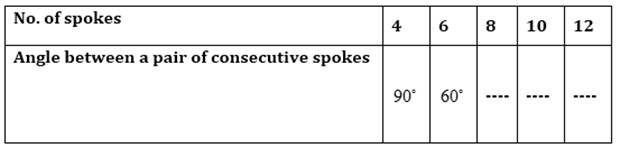Ans.

Here the number of spokes are increasing and the angle between a pair of consecutive spokes is decreasing. So, it is a inverse proportion and angle at the centre of a circle is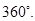When the number of spokes is 8, then angle between a pair of consecutive spokes =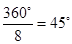When the number of spokes is 10, then angle between a pair of consecutive spokes=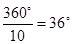When the number of spokes is 12, then angle between a pair of consecutive spokes=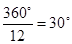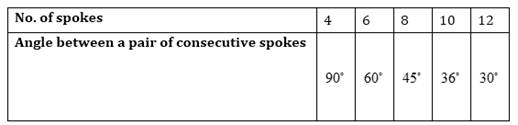(i) Yes, the number of spokes and the angles formed between a pair of consecutive spokes is in inverse proportion.

(ii) When the number of spokes is 15, then angle between a pair of consecutive spokes=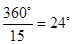.

(iii) The number of spokes would be needed =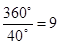###### 4. If a box of sweets is divided among 24 children, they will get 5 sweets each. How many would each get, if the number of the children is reduced by 4?

Ans.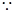Each child gets = 5 sweets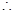24 children will get 24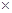5 = 120 sweets

Total number of sweets = 120

If the number of children is reduced by 4, then children left = 24 – 4 = 20

Now each child will get sweets =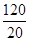= 6 sweets

NCERT Solutions for Class 8 Maths Exercise 13.2

###### 5. A farmer has enough food to feed 20 animals in his cattle for 6 days. How long would the food last if there were 10 more animals in his cattle?

Ans. Let the number of days be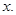Total number of animals = 20 + 10 = 30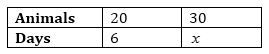Here the number of animals and the number of days are in inverse proportion.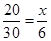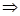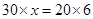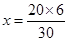= 4

Hence the food will last for four days.

NCERT Solutions for Class 8 Maths Exercise 13.2

###### 6. A contractor estimates that 3 persons could rewire Jasminder’s house in 4 days. If, he uses 4 persons instead of three, how long should they take to complete the job?

Ans. Let time taken to complete the job be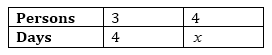Here the number of persons and the number of days are in inverse proportion.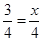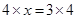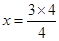= 3 days

Hence they will complete the job in 3 days.

NCERT Solutions for Class 8 Maths Exercise 13.2

###### 7. A batch of bottles was packed in 25 boxes with 12 bottles in each box. If the same batch is packed using 20 bottles in each box, how many boxes would be filled?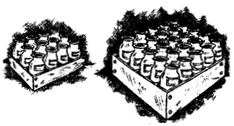Ans. Let the number of boxes be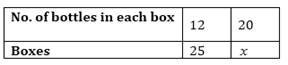Here the number of bottles and the number of boxes are in inverse proportion.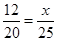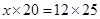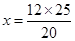= 15

Hence 15 boxes would be filled.

NCERT Solutions for Class 8 Maths Exercise 13.2

###### 8. A factory requires 42 machines to produce a given number of articles in 63 days. How many machines would be required to produce the same number of articles in 54 days?

Ans. Let the number of machines required be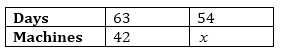Here the number of machines and the number of days are in inverse proportion.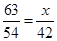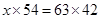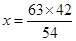= 49

Hence 49machineswould be required.

NCERT Solutions for Class 8 Maths Exercise 13.2

###### 9. A car takes 2 hours to reach a destination by travelling at the spe^ed of 60 km/hr. How long will it take when the car travels at the speed of 80 km/hr?

Ans. Let the number of hours be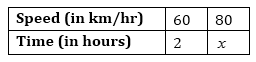Here the speed of car and time are in inverse proportion.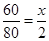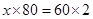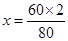=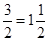hrs.

Hence the car will take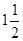hours

to reach its destination.

NCERT Solutions for Class 8 Maths Exercise 13.2

###### 10. Two persons could fit new windows in a house in 3 days.

(i) One of the persons fell ill before the work started. How long would the job take now?

(ii) How many persons would be needed to fit the windows in one day?

Ans. (i) Let the number of days be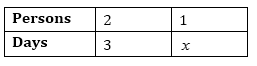Here the number of persons and the number of days are in inverse proportion.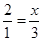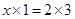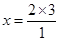= 6 days

(ii)  Let the number of persons be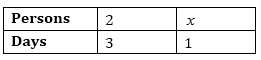Here the number of persons and the number of days are in inverse proportion.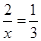= 6 persons

NCERT Solutions for Class 8 Maths Exercise 13.2

###### 11. A school has 8 periods a day each of 45 minutes duration. How long would each period be, if the school has 9 periods a day, assuming the number of school hours to be the same?

Ans. Let the duration of each period be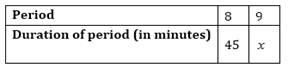Here the number of periods and the duration of periods are in inverse proportion.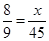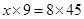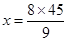= 40 minutes

Hence duration of each period would be 40 minutes.

## NCERT Solutions for Class 8 Maths Exercise 13.2

NCERT Solutions Class 8 Mathematics PDF (Download) Free from myCBSEguide app and myCBSEguide website. Ncert solution class 8 Mathematics includes text book solutions from Class 8 Maths Book . NCERT Solutions for CBSE Class 8 Maths have total 16 chapters. 8 Maths NCERT Solutions in PDF for free Download on our website. Ncert class 8 solutions PDF and Maths ncert class 8 PDF solutions with latest modifications and as per the latest CBSE syllabus are only available in myCBSEguide.

## CBSE app for Class 8

To download NCERT Solutions for class 8 Social Science, Computer Science, Home Science,Hindi ,English, Maths Science do check myCBSEguide app or website. myCBSEguide provides sample papers with solution, test papers for chapter-wise practice, NCERT solutions, NCERT Exemplar solutions, quick revision notes for ready reference, CBSE guess papers and CBSE important question papers. Sample Paper all are made available through the best app for CBSE students and myCBSEguide website.Test Generator

Create question paper PDF and online tests with your own name & logo in minutes.myCBSEguide

Question Bank, Mock Tests, Exam Papers, NCERT Solutions, Sample Papers, Notes

### 15 thoughts on “NCERT Solutions for Class 8 Maths Exercise 13.2”

1. I LIKE THIS SITE . THIS SO NICE SITE ??

2. Thanks for this by this I can make my homework easy…..

3. Nice work you do …..?????!!!?

4. This app is so much good useful

5. Good!

6. Nice

7. Nice website

8. Good but it is direct and inverse proportion not linear equation.

9. Nice ???

10. Very useful app..love this app??

11. This site is very best

12. Nice work

13. I love this app thank you so much

14. Thank u so much for this

15. This helps me a lot